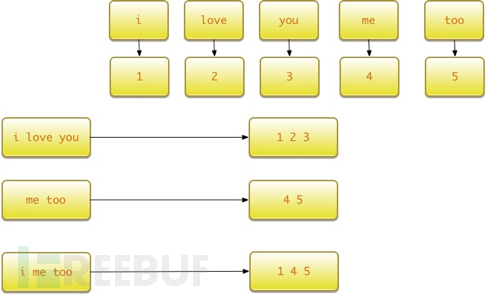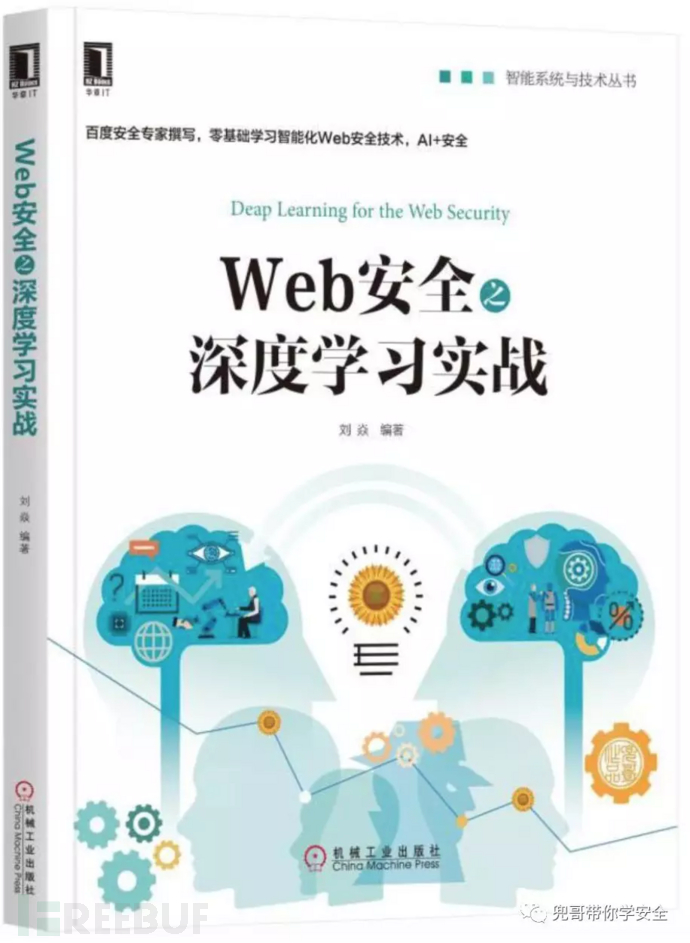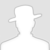FreeBuf.COM网络安全行业门户，每日发布专业的安全资讯、技术剖析。FreeBuf+小程序

NLP系列之词袋模型和TFIDF模型
2018-03-31 16:00:09

## 词袋模型

• 词集模型：单词构成的集合，集合自然每个元素都只有一个，也即词集中的每个单词都只有一个。

• 词袋模型：在词集的基础上如果一个单词在文档中出现不止一次，统计其出现的次数（频数）。

``````from sklearn.feature_extraction.text import CountVectorizer
``````

``````vectorizer = CountVectorizer(min_df=1)

vectorizer

CountVectorizer(analyzer=...'word', binary=False, decode_error=...'strict',

dtype=<... 'numpy.int64'>, encoding=...'utf-8', input=...'content',

lowercase=True, max_df=1.0, max_features=None, min_df=1,

ngram_range=(1, 1), preprocessor=None, stop_words=None,

strip_accents=None, token_pattern=...'(?u)\\b\\w\\w+\\b',

tokenizer=None, vocabulary=None)
``````

``````corpus = [

...     'This is the first document.',

...     'This is the second second document.',

...     'And the third one.',

...     'Is this the first document?',

... ]

X = vectorizer.fit_transform(corpus)

X

<4x9 sparse matrix of type '<... 'numpy.int64'>'

with 19 stored elements in Compressed Sparse ... format>
``````

``````>>> vectorizer.get_feature_names() == (

...     ['and', 'document', 'first', 'is', 'one',

...      'second', 'the', 'third', 'this'])

True
``````

``````>>> X.toarray()

array([[0, 1, 1, 1, 0, 0, 1, 0, 1],

[0, 1, 0, 1, 0, 2, 1, 0, 1],

[1, 0, 0, 0, 1, 0, 1, 1, 0],

[0, 1, 1, 1, 0, 0, 1, 0, 1]]...)
``````

vocabulary=vectorizer.vocabulary_

`````` new_vectorizer = CountVectorizer(min_df=1, vocabulary=vocabulary)
``````

CountVectorize函数比较重要的几个参数为：

• decode_error，处理解码失败的方式，分为‘strict’、‘ignore’、‘replace’三种方式。

• strip_accents，在预处理步骤中移除重音的方式。

• max_features，词袋特征个数的最大值。

• stop_words，判断word结束的方式。

• max_df，df最大值。

• min_df，df最小值 。

• binary，默认为False，当与TF-IDF结合使用时需要设置为True。

## TF-IDF模型

TF-IDF的主要思想是，如果某个词或短语在一篇文章中出现的频率TF(Term Frequency，词频)，词频高，并且在其他文章中很少出现，则认为此词或者短语具有很好的类别区分能力，适合用来分类。TF-IDF实际上是：TF * IDF。TF表示词条在文档d中出现的频率。IDF（inverse document frequency，逆向文件频率）的主要思想是：如果包含词条t的文档越少，也就是n越小，IDF越大，则说明词条t具有很好的类别区分能力。如果某一类文档C中包含词条t的文档数为m，而其他类包含t的文档总数为k，显然所有包含t的文档数n=m+k，当m大的时候，n也大，按照IDF公式得到的IDF的值会小，就说明该词条t类别区分能力不强。但是实际上，如果一个词条在一个类的文档中频繁出现，则说明该词条能够很好代表这个类的文本的特征，这样的词条应该给它们赋予较高的权重，并选来作为该类文本的特征词以区别与其他类文档。

``````from sklearn.feature_extraction.text import TfidfTransformer

transformer = TfidfTransformer(smooth_idf=False)

transformer

TfidfTransformer(norm=...'l2', smooth_idf=False, sublinear_tf=False, use_idf=True)
``````

TF-IDF模型通常和词袋模型配合使用，对词袋模型生成的数组进一步处理：

``````>>> counts = [[3, 0, 1],

...           [2, 0, 0],

...           [3, 0, 0],

...           [4, 0, 0],

...           [3, 2, 0],

...           [3, 0, 2]]

...

>>> tfidf = transformer.fit_transform(counts)

>>> tfidf

<6x3 sparse matrix of type '<... 'numpy.float64'>'     with 9 stored elements in Compressed Sparse ... format>

>>> tfidf.toarray()

array([[ 0.81940995,  0.        ,  0.57320793],

[ 1.        ,  0.        ,  0.        ],

[ 1.        ,  0.        ,  0.        ],

[ 1.        ,  0.        ,  0.        ],

[ 0.47330339,  0.88089948,  0.        ],

[ 0.58149261,  0.        ,  0.81355169]])
``````

## 词汇表模型

``````tf.contrib.learn.preprocessing.VocabularyProcessor (

max_document_length,

min_frequency=0,

vocabulary=None,

tokenizer_fn=None)
``````

• max_document_length:，文档的最大长度。如果文本的长度大于最大长度，那么它会被剪切，反之则用0填充。

• min_frequency，词频的最小值，出现次数小于最小词频则不会被收录到词表中。

• vocabulary，CategoricalVocabulary 对象。

• tokenizer_fn，分词函数。

``````x_text =[

'i love you',

'me too'

]
``````

``````vocab_processor = learn.preprocessing.VocabularyProcessor(max_document_length)

vocab_processor.fit(x_text)

print next(vocab_processor.transform(['i me too'])).tolist()

x = np.array(list(vocab_processor.fit_transform(x_text)))

print x
``````

`````` [[1 2 3 0]

[4 5 0 0]]
``````

'i me too'这句话编码的结果为：

``````[1, 4, 5, 0]
``````# 机器学习 # NLP

+ 收入我的专辑\
• 0 文章数
• 0 评论数
• 0 关注者